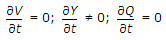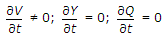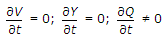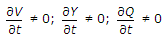# Civil Engineering - Hydraulics

### Exercise :: Hydraulics - Section 3

6.

Which one of the following equation is applicable to unsteady flow in open channels :

 A.B.C.D.Explanation:

No answer description available for this question. Let us discuss.

7.

Dimensions of the dynamic viscosity (μ) are

 A. MLT-2 B. M-1L-1T-1 C. ML-1T-2 D. ML-1T-1.

Explanation:

No answer description available for this question. Let us discuss.

8.

To avoid vapourisation, pipe lines are laid over the ridge so that these are above the hydraulic gradient line, not more than

 A. 2.4 m B. 6.4 m C. 10.0 m D. 5.0 m.

Explanation:

No answer description available for this question. Let us discuss.

9.

A jet projected at an angle of 45θ, 40 m from the foot of a vertical column, just reaches the top of the column. The height of the column is

 A. 15 m B. 20 m C. 30 m D. 40 m E. 60 m.

Explanation:

No answer description available for this question. Let us discuss.

10.

If Cv, Cc, Cd and Cr are the hydraulic coefficients of an orifice, then

 A. Cd = Cc . Cv B. Cr = 1 + Cv2/Cd C. Cv = Cc + Cd D. Cc = Cv/Cd